# Question about the MBD of MPC5744P

cancel
Showing results for
Show  only  | Search instead for
Did you mean:

## Question about the MBD of MPC5744P

609 ViewsContributor IV

hello，

now  i am studying the demo of "VF_control_MP574xP"

but , I have a question about the module"ComputeElectricAngle"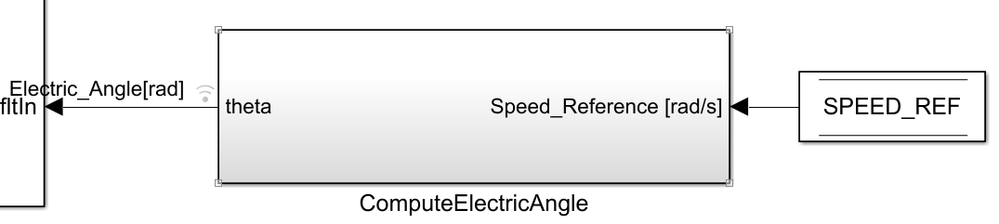In this module, the f32C1 is configured with "(2*pi/60)*PP*Nmax*(C_LST/2/pi)*(2^31)"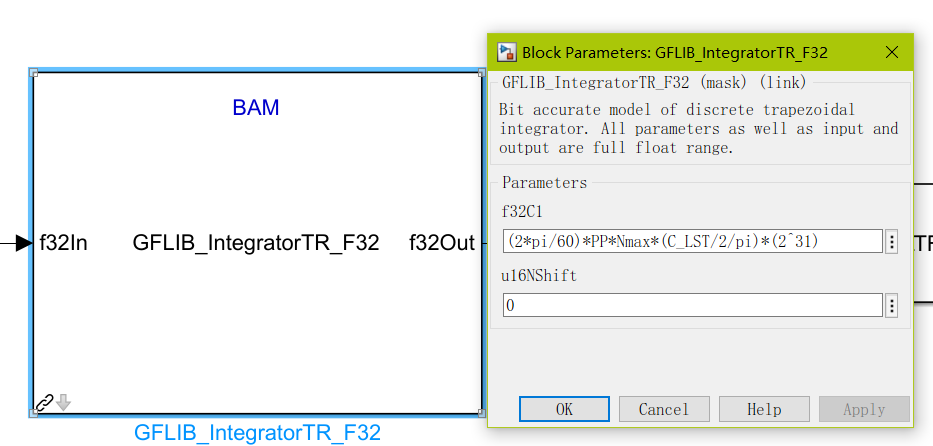the input of integrator is "Speed_Reference/(Nmax*(2*pi/60))"

the output of integrator multiply by pi is electric_Angle.

but the manul of the integrator is no same as above forms.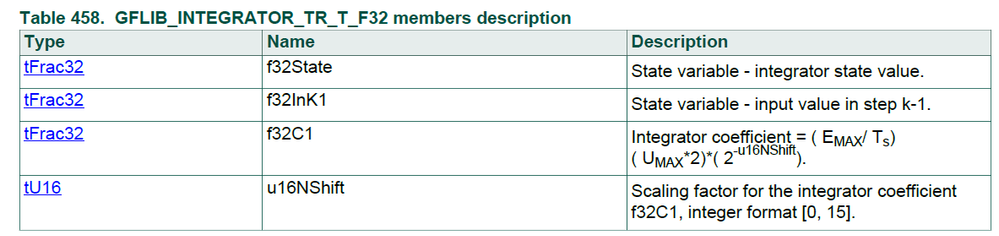Could anyone help to solve my confuse?

thank you very much.

best regards.

hao

2 Replies
492 ViewsNXP Employee

Hi hao xue

Typically in PMSM, you need to transform feed the rotor position with respect to phase A, to perform the Park transformations (direct & inverse), and that angle needs to be provided in a measurement using used by sine/cosine functions you are using.

In the case of the AMMCLIB sine/cosine, we need to provide the angle in (-pi, pi) range.

In the open-loop, we take the value to the speed reference (rpm or rad/s) and we need to transform that into angle information.

Furthermore, later on, you need to consider the computation expansiveness of various implementation - hence you need to perform this transformation rad/s -> rad as fast as possible. Since these computations are performed on the single-precision floating-point numbers then you need to eliminate any potential function calls to floating-point functions library like mod().

So, to come back to your questions: I'm using the integrator to perform a mathematical trick. I'm attaching a model below - which compares 2 methods of angle computation. Both methods are doing the same thing. But in code generation, the methods with AMMCLIB blocks will run faster due to the avoidance of expensive floating-point functions calls from the standard floating-point library (see this subject: How to Squeeze Every Last Drop of Performance Out of SIMULINK Models with AMMCLIB  )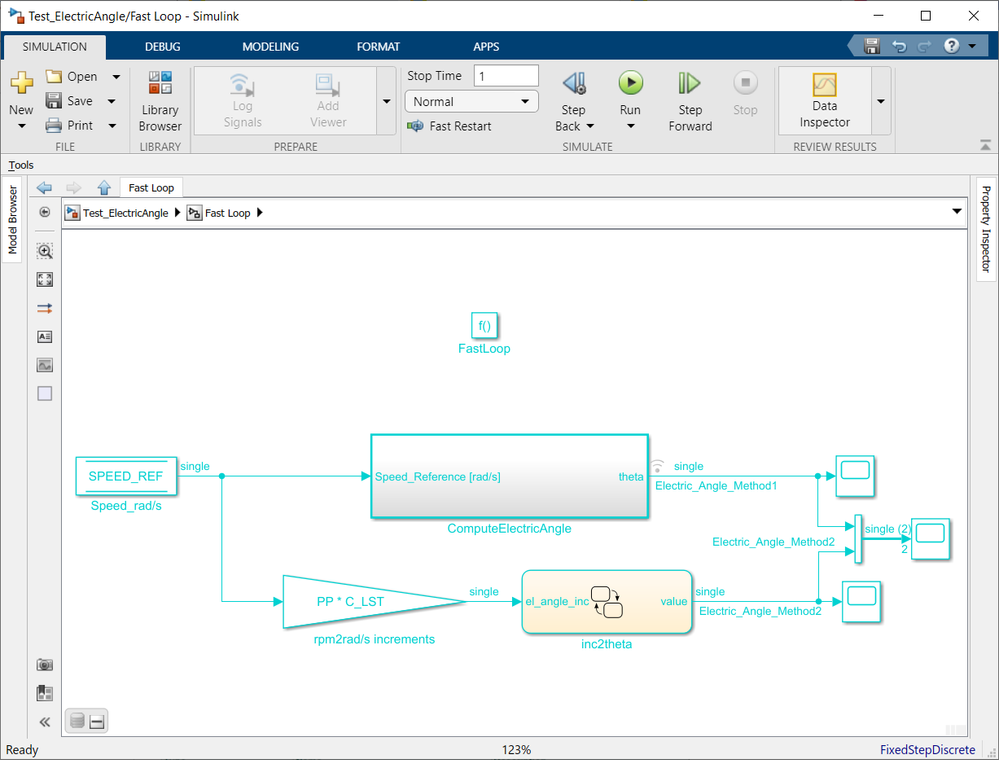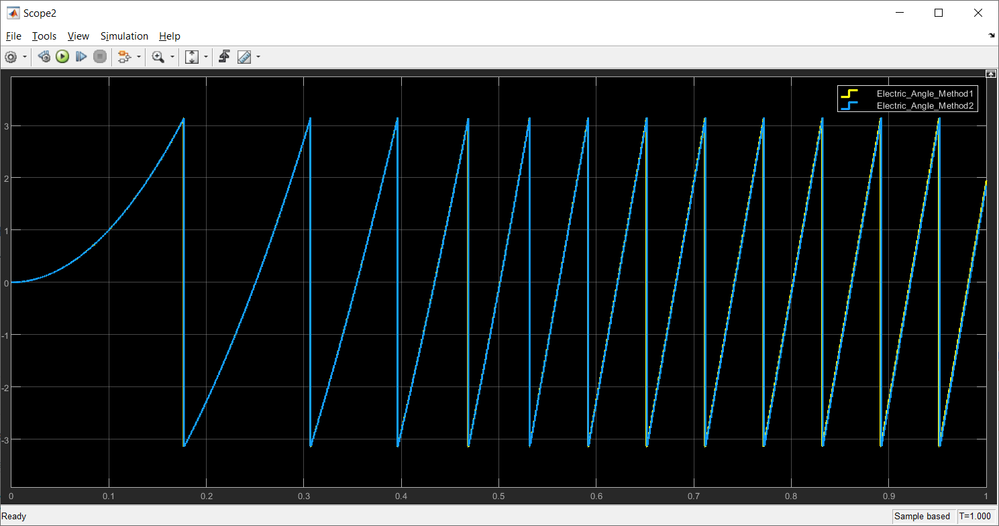The resolution fo the first method is better than the second - one of the aspects being the maximum speed that can be obtained with the motor. Instead of integrating the entire numerical domain, you can limit the range by specifying the maximum speed that your motor is capable of.

The f32C1 integrator gain is computed so that the integrator will manage 2 mathematical computations:

- wrap around in +/- range due to the mathematical properties of additions on fixed-point calculations.

In fact, the second aspect - the wrap-around is what interested me the most, because then I can just convert the integrator result to a float number in the range of -1:1 and simply multiply it by pi. This way I know for sure is not going to feed wrong data into the sine/cosine function.

Please use the model attached, perform some simulations, check with scopes various signals and I'm pretty sure you will figure all the details.

Hope this helps!

Daniel

491 ViewsContributor IV